# 我的机器学习课笔记 #2-线性模型

[TOC]## 线性回归

$$f(x_i)=wx_i+b，f(x)\approx y_i$$

$$argmin(\sum_{i=1}^{m}(f(x_i)-y_i)^2)$$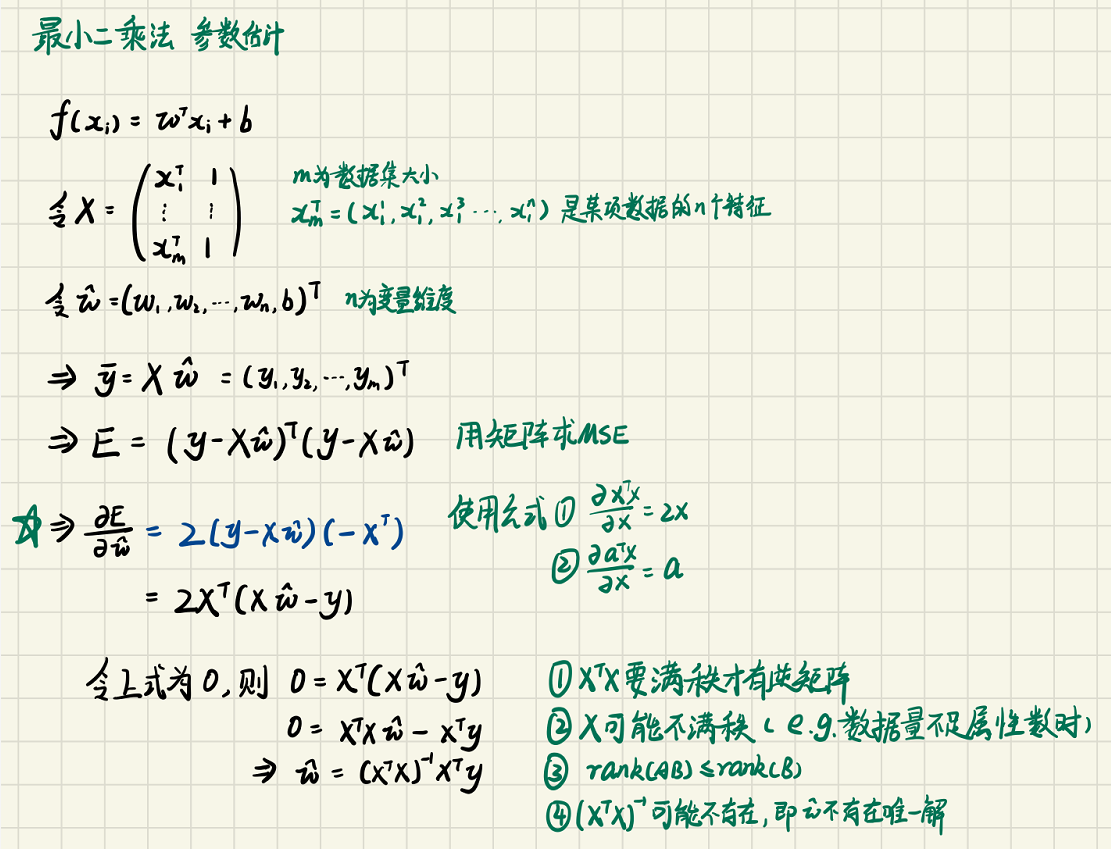$$广义线性模型： y=g^{-1}(w^Tx+b)$$

## 线性回归改进——正则化

L1范数就是$||w||1=\sum^{d}{i=1}|w_i|$，即向量w所有元素的绝对值的和

L2范数就是$||w||2=\sum^{d}{i=1}w_i^2$，即向量w所有元素的平方的和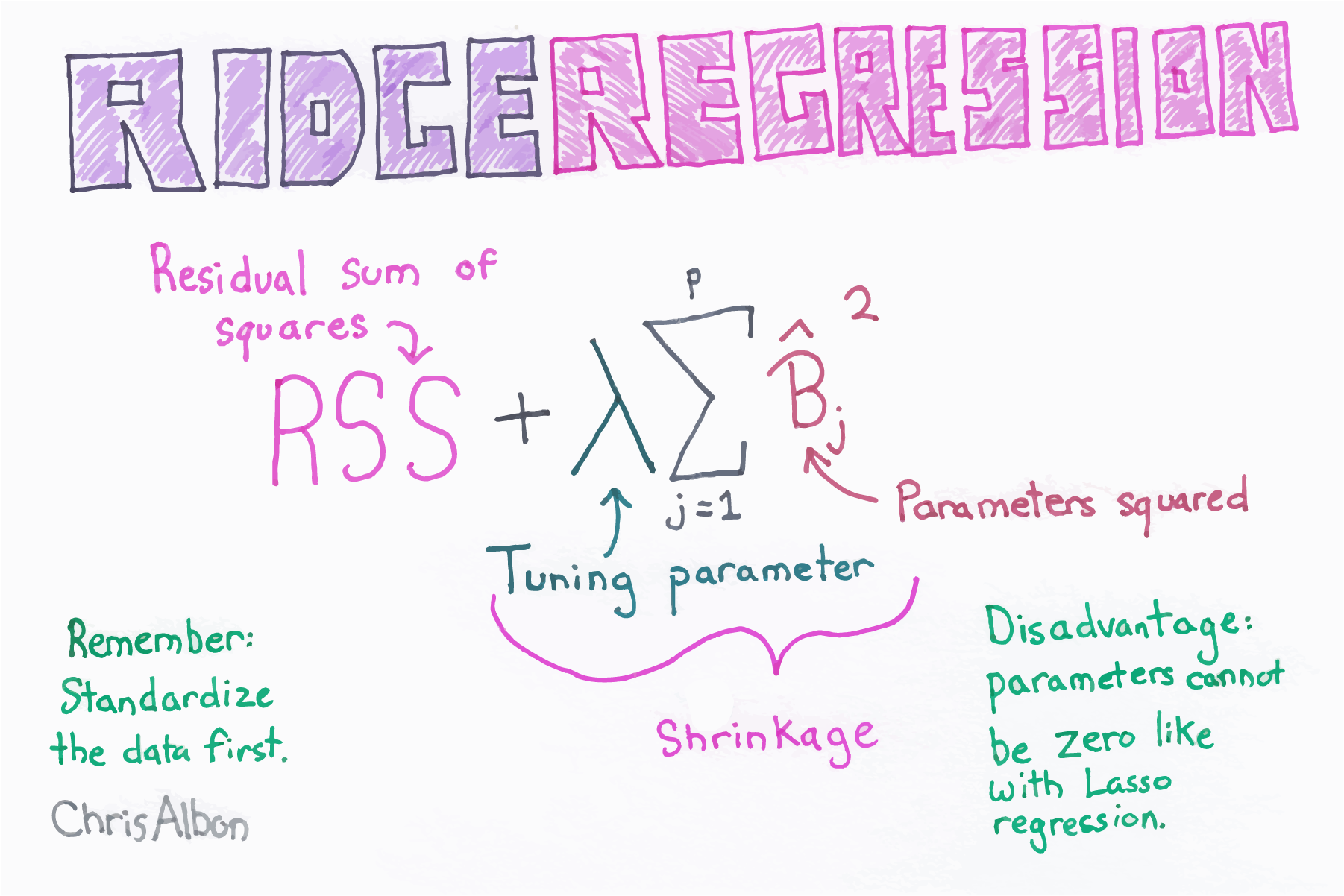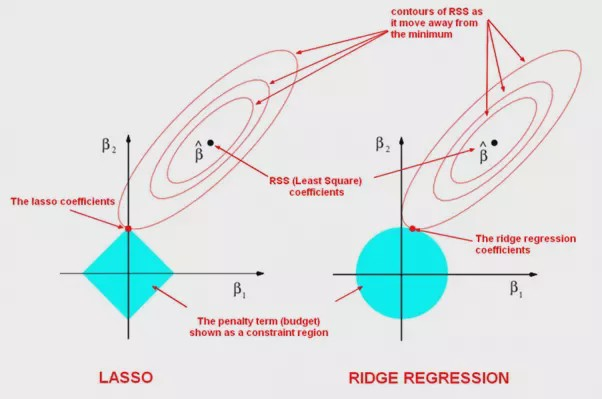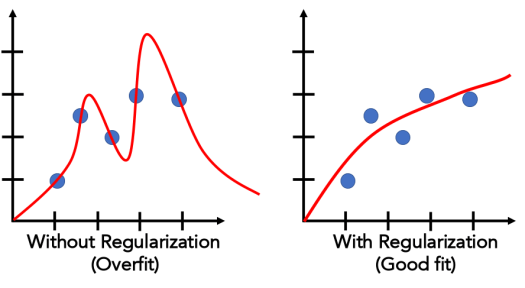## 线性分类（对数几率回归、逻辑回归）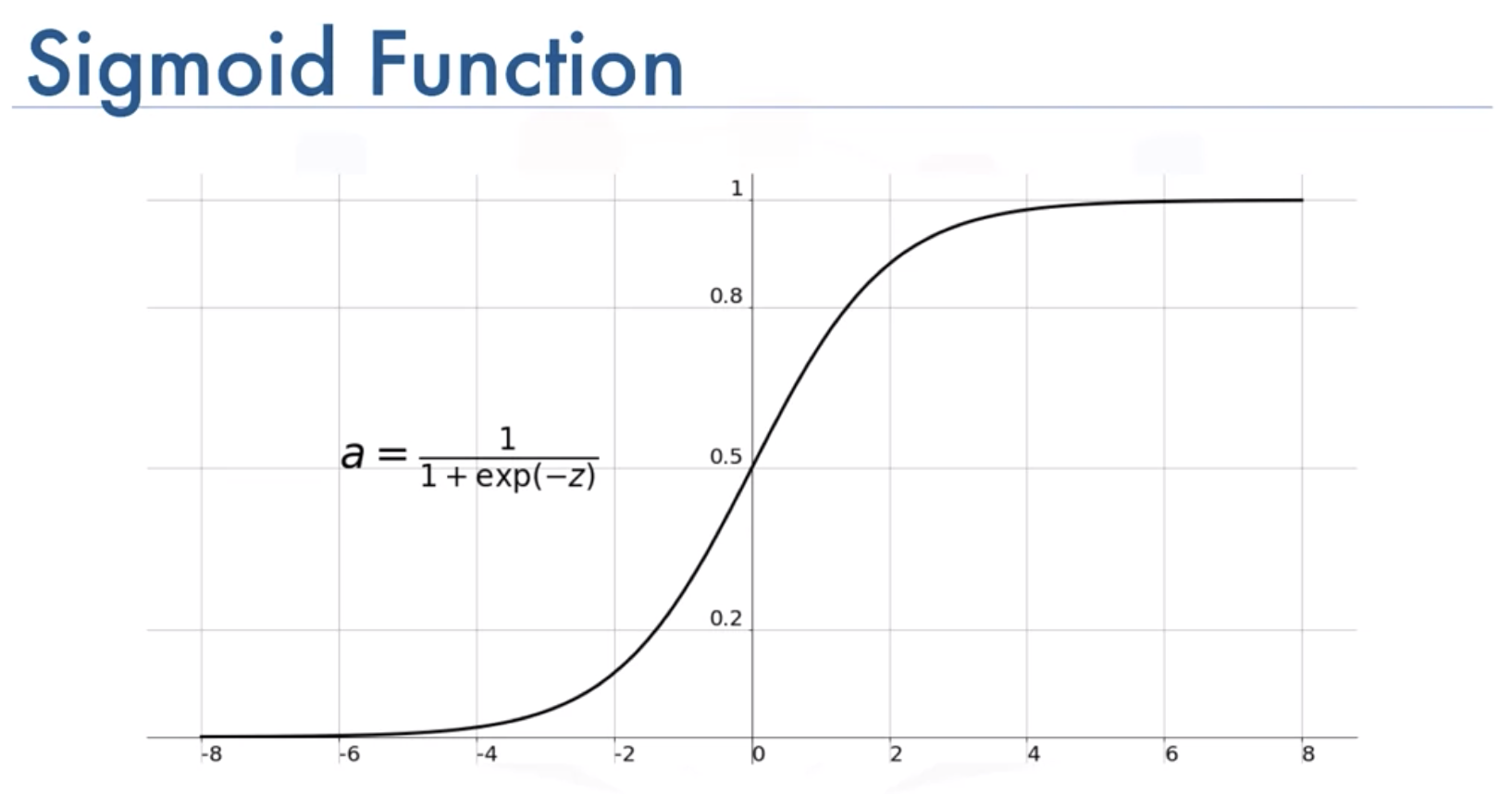MSEloss的值用E表示，用标签 $y_i$减去$\sigma$出来的概率，再求平方，然后对loss求偏导如公式所示，当$\sigma$接近$y_i$时，$\sigma$和$1-\sigma$总有一个趋近于0，此时梯度消失。举个例子：当y=1时，预测出来σ=0.00001，此时距离真实值很远，应该梯度很大，然而梯度接近0。$$p(y=1|x)=sigmoid(w^Tx+b)$$
$$p(y=0|x)=1-sigmoid(w^Tx+b)$$
$$Loss(w,b)=\sum^m_{i=1}{\ln{p(y_i|x_i;w, b)}} 其中x_i,y_i是一对数据集标注$$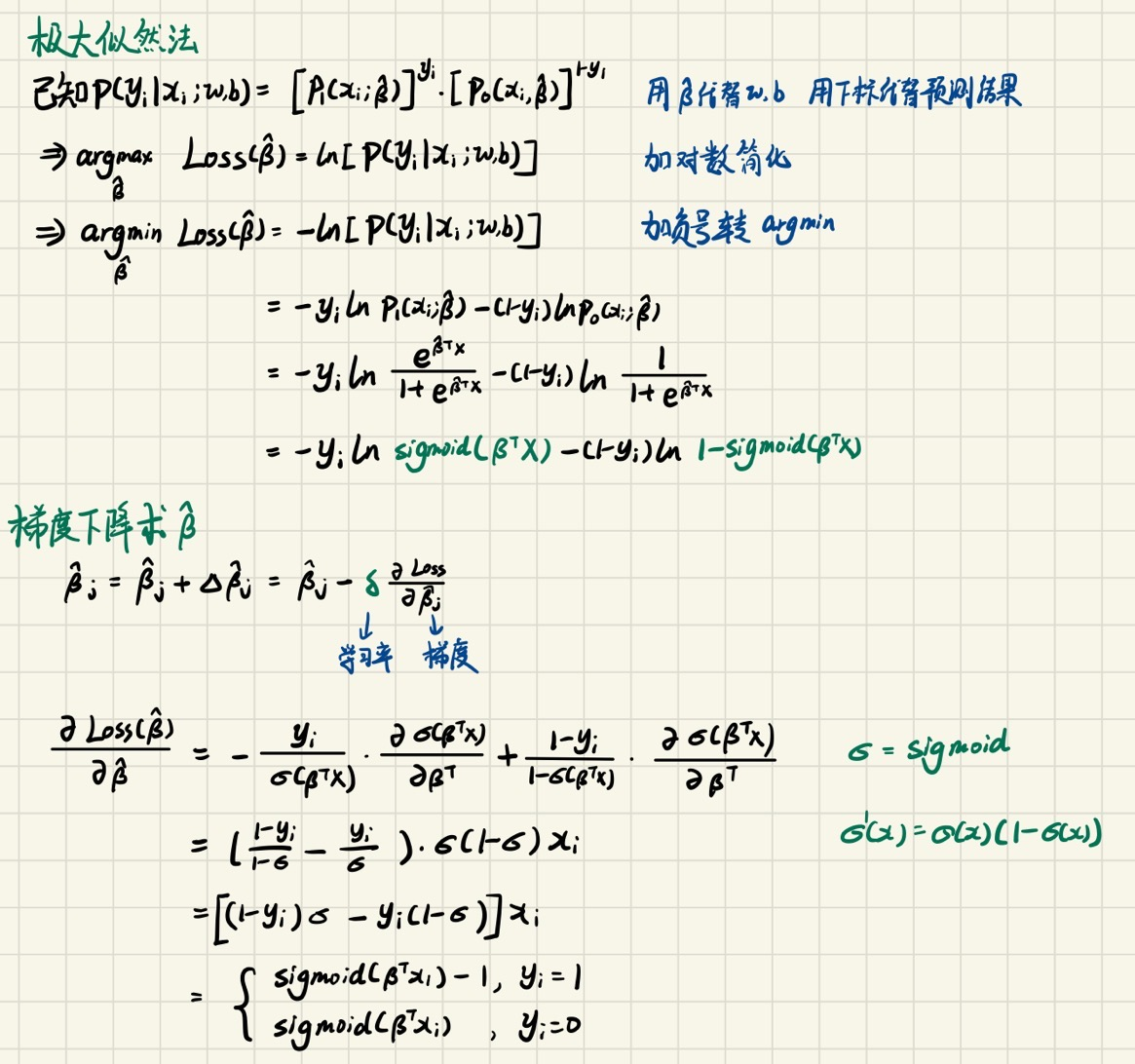## 多分类学习

• ovo，就是把N个类别两两配对，训练 $\frac{N(N-1)}{2}$个二分类器，然后通过这些二分类器的结果进行投票得出最终预测值
• ovr，就是训练N个二分类器，每次都选取一个类别作为正例，其余为负例，这样只用训练N个但是数据不均衡
• mvm，每次选择若干个类作为正例，若干个类作为负例。这种选取有很多种方式，在这里不详细介绍。

Ridge Regression (chrisalbon.com)

AI 面试高频问题: 为什么二分类不用 MSE 损失函数？-技术圈 (proginn.com)